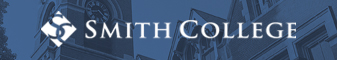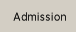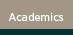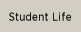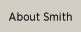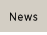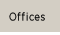The Spinelli Center for Quantitative Learning often holds a set of workshops for those interested in reviewing some basic math skills that arise when studying for the Graduate Record Exams (GRE) or the MTEL (Massachusetts Teacher Exam for Licensure). This is not a GRE or MTEL review, but we review topics that are included on the GRE and typically show up on standardized tests.

For a list of topics you should review, see below. We recommend that you buy a book of practice problems and come to the Spinelli Center once a week to check in with a tutor about your math practice and ask questions.

Arithmetic I (symbols, types of numbers, factors, order of operations, fractions)

Arithmetic II (decimals, ratios, proportions, percents, exponents, roots, absolute value)

Algebra I (terms, factoring, linear equations, reverse order of operations, quadratic equations, inequalities)

Algebra I (terms, factoring, linear equations, reverse order of operations, quadratic equations, inequalities)

Algebra II (coordinate geometry, slopes, parallel lines, linear equations, simultaneous equations)

Geometry I (lines, angles, polygons, triangles, Pythagorean theorem, rectangles)

Geometry II (parallelograms, trapezoids, circles, arc length, 3-D solids)

Data analysis I (mean, median, counting, frequency, probability, sequences,)

Data analysis II (factorials, permutations, combinations, group counting)What to Expect

Math Success at Smith

Online Resources

Courses